Search IntMath
Close

450+ Math Lessons written by Math Professors and Teachers

5 Million+ Students Helped Each Year

1200+ Articles Written by Math Educators and Enthusiasts

Simplifying and Teaching Math for Over 23 Years

# Units: Calories and kilograms

By Murray Bourne, 14 Dec 2018

Two related stories about units came my way recently.

### (a) Calorie or calorie?

Reader goldierocks recently commented on an article I wrote some years ago (Google calculator - handy, but not always correct) when Google began incorporating calculator answers into search. The calculator had scientific options (trigonometric calculations, logs, and so on) and was a good tool, especially as it was free.

However, it didn't handle large number calculations well - the inevitable product of computers thinking in binary digits, while we humans ask it to calculate things in the decimal system.

My article pointed out what some of those large number calculation errors were, and I pointed to a longer article with more detail.

Back to the goldierocks comment, where (s)he said:

Google calculator can be hopelessly wrong. For energy calculations it can be out by a factor of 1000 times (e.g. calorie to kilojoules - they have confused calories and kilocalories)

I did a bit more investigation before I replied.

On this Convert calories to kJ page by CheckYourMath, which provides a calculator for conversions in both directions, it says:

A calorie is measured as the quantity of heat required to raise the temperature of 1 gram of water by 1°C from a standard initial temperature at a pressure of 1 atmosphere. A calorie is also called a "small calorie" or "gram calorie".

For most people, the first thing that comes to mind when we hear "calorie" is the size of our wasteline and the need to cut food consumption. (The word "calorie" is derived from the French word "calor" which means "heat").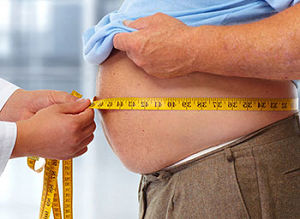This Britannica article on Calorie points out the (small "c") calorie ranges from 4.1855 joules to 4.19002 joules (it varies depending on the ambient temperature and pressure), while the (upper case "C") Calorie (or "kilogram calorie") used by food scientists, is really a kilocalorie, or 1,000 calories. For example:

If a peach is listed as having 40 Calories, this indicates that that peach has actually 40,000 calories

The CheckYourMath page correctly used the lower-case "c".

So as I said to goldierocks, the Google calculator is addressing their most likely audience of obesity worriers - not the smaller number of science majors. However, the search result should point out they are using "food Calories" and use upper-case "C", wherever that is the case .

#### Interesting search results

I noticed some interesting differences in the Google results with what seems like almost identical search terms.

(1) 1 calorie in kj

Here I'm using lower-case "j" in "kj". The result (in the drop-down list in the address bar, before going on to the search results page) is the "food Calorie" case: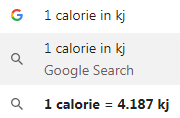(2) 1 calorie in kJ

When I do the same thing using upper-case "J" as in "kJ", it appears to assume I have more scientific intent and so gives the "small c" answer in the address bar. Note the icon that indicates it's giving the answer using the "scientific calculator":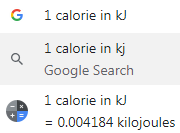(3) Opening the actual search result page

This time, it gives a "kilocalorie to kilojoules" result: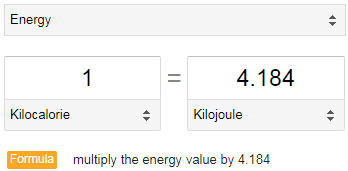### (b) The kilogram is changing

The kilogram is one of the base units in the metric system, along with the meter, second, Ampere, Kelvin, Mole, candela.

For over 200 years, the "standard kilogram", used to compare and calibrate scales all over the world, has been a metalic cylinder kept in France. In Kilogram definition to be changed based on Planck’s constant we learn the problem with this cylinder is that over time, it's weight has actually dropped by around 50 µg, about the mass of an eyelash) and that means the precision of anything that is compared to it, becomes inaccurate over time.

In the Vox article, The world just redefined the kilogram they point out there are several copies of the "kilogram cylinder", including in the US. Instead of (sensibly) using it as-is and producing metric scales, they do a conversion (in the US, 1 pound is defined as 0.45359237 kilograms. A foot is defined as 0.3048 meters.)

They also describe the Kibble balance, a machine that can make use of Planck's constant to very precisely weigh objects.

As a result of the recent meeting, the Resolutions (PDF) of the General Conference on Weights and Measures state:

The kilogram, symbol kg, is the SI unit of mass. It is defined by taking the fixed numerical value of the Planck constant h to be 6.626 070 15 × 10–34 when expressed in the unit J s, which is equal to kg m2 s–1, where the metre and the second are defined in terms of c and [the caesium frequency] ΔvCs.

#### Irony

There is an image on the Wikipedia Kilogram page of Le Grand K, the cylinder made of platinum-iridium alloy which has represented the kg since 1799.

Strangely, it uses an inch ruler for size comparison!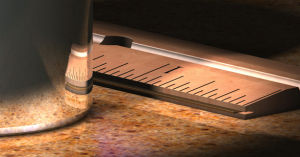See the 1 Comment below.

### One Comment on “Units: Calories and kilograms”

1. Stephen says:

Yes, the Americans just can't leave that ancient Imperial system be. You'd think the country that claims to be the most advanced technically would have embraced the superior metric system long ago.

### Comment Preview

HTML: You can use simple tags like <b>, <a href="...">, etc.

To enter math, you can can either:

1. Use simple calculator-like input in the following format (surround your math in backticks, or qq on tablet or phone):
a^2 = sqrt(b^2 + c^2)
(See more on ASCIIMath syntax); or
2. Use simple LaTeX in the following format. Surround your math with $$ and $$.
$$\int g dx = \sqrt{\frac{a}{b}}$$
(This is standard simple LaTeX.)

NOTE: You can mix both types of math entry in your comment.